Practice Test: Arithmetic Progressions

# Practice Test: Arithmetic Progressions

Test Description

## 25 Questions MCQ Test Mathematics (Maths) Class 10 | Practice Test: Arithmetic Progressions

Practice Test: Arithmetic Progressions for Class 10 2022 is part of Mathematics (Maths) Class 10 preparation. The Practice Test: Arithmetic Progressions questions and answers have been prepared according to the Class 10 exam syllabus.The Practice Test: Arithmetic Progressions MCQs are made for Class 10 2022 Exam. Find important definitions, questions, notes, meanings, examples, exercises, MCQs and online tests for Practice Test: Arithmetic Progressions below.
Solutions of Practice Test: Arithmetic Progressions questions in English are available as part of our Mathematics (Maths) Class 10 for Class 10 & Practice Test: Arithmetic Progressions solutions in Hindi for Mathematics (Maths) Class 10 course. Download more important topics, notes, lectures and mock test series for Class 10 Exam by signing up for free. Attempt Practice Test: Arithmetic Progressions | 25 questions in 25 minutes | Mock test for Class 10 preparation | Free important questions MCQ to study Mathematics (Maths) Class 10 for Class 10 Exam | Download free PDF with solutions
 1 Crore+ students have signed up on EduRev. Have you?
Practice Test: Arithmetic Progressions - Question 1

### If the sum of first n terms of an AP be 3n2 – n and it's common difference is 6, then its first term is :

Detailed Solution for Practice Test: Arithmetic Progressions - Question 1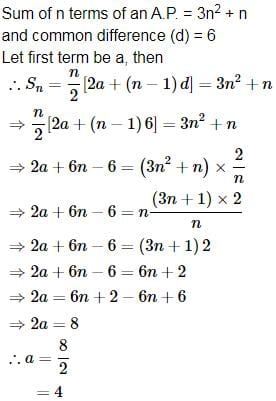Practice Test: Arithmetic Progressions - Question 2

### If 7th and 13th terms of an A.P. be 34 and 64, respectively, then it's 18th term is :

Practice Test: Arithmetic Progressions - Question 3

### The sum of all 2-digit odd positive numbers is :

Detailed Solution for Practice Test: Arithmetic Progressions - Question 3

Here a = 11 and d = 2, tn= 99, n = ?
Sum of the n terms = (n/2)[2a+(n -1)d]
But tn = a + (n -1)d
⇒ 99 = 11+ (n-1)2
⇒ 99 -11 = (n-1)2
⇒ 88/2 = (n-1)
∴ n = 45.
subsitute n = 45  in sum of the n terms we obtain
⇒ s45 = (45/2)(2×11 + (45 -1)2)
⇒ s45 = (45/2)(110)
⇒ s45 = 45×55.
⇒  s45 = 2475.
∴ sum of all two digit odd positive numbers = 2475.

Practice Test: Arithmetic Progressions - Question 4

The fourth term of an A.P. is 4. Then the sum of the first 7 terms is :

Detailed Solution for Practice Test: Arithmetic Progressions - Question 4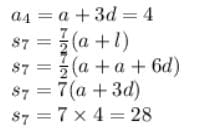Practice Test: Arithmetic Progressions - Question 5

In an A.P., s1 = 6, s7 = 105, then sn : sn-3 is same as :

Practice Test: Arithmetic Progressions - Question 6

In an A.P. s3 = 6, s6 = 3, then it's common difference is equal to :

Detailed Solution for Practice Test: Arithmetic Progressions - Question 6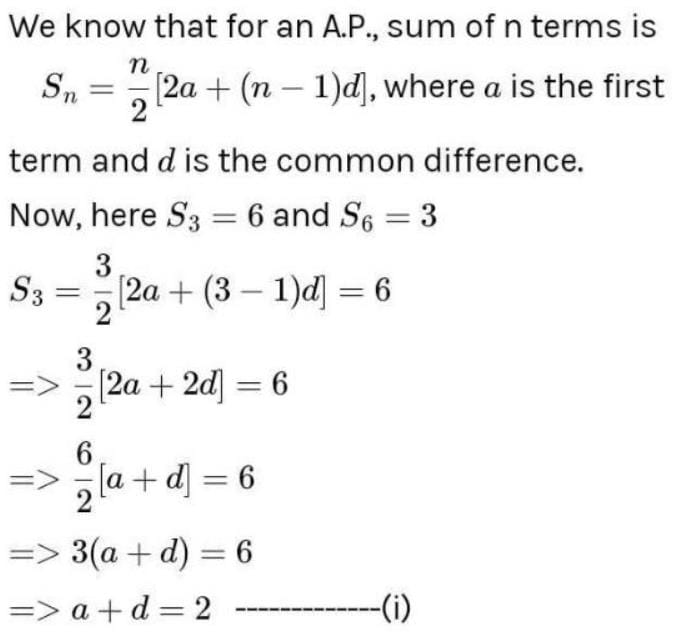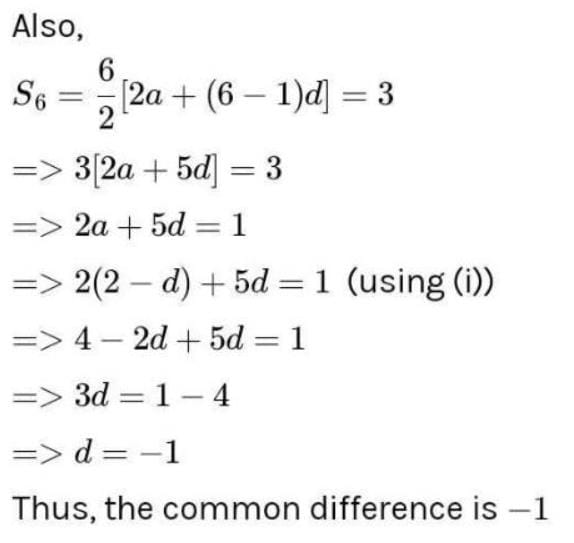Practice Test: Arithmetic Progressions - Question 7

The number of terms common to the two A.P. s 2 + 5 + 8 + 11 + ...+ 98 and 3 + 8 + 13 + 18 +...+198

Detailed Solution for Practice Test: Arithmetic Progressions - Question 7

For first A.P
2+5+8+11+......+98
a=2,an​=98,d=3
an​=a+(n−1)d
98=2+(n−1)3
98=2+3n−3
3n=99
n=33
Number of term =33
For first A.P
3+8+13+18+......+198
a=3,an​=198,d=5
an​=a+(n−1)d
198=3+(n−1)5
198=3+5n−5
5n=200
n=40
No of terms =40
Common terms=40−33=7

Practice Test: Arithmetic Progressions - Question 8

(p + q)th and (p – q)th terms of an A.P. are respectively m and n. The pth term is :

Detailed Solution for Practice Test: Arithmetic Progressions - Question 8

l=a+(n-1)d
(p+q)th term is m
m=a+(p+q-1)d
m=a+pd+qd-d  ….1
(p-q)th term is n
n=a+(p-q-1)d
n=a+pd-qd-d   ….2
Adding 1 and 2
m+n=2a+2pd-2d
m+n=2(a+pd-d)
½(m+n=a+(p-1)d
So pth term is ½(m+n)

Practice Test: Arithmetic Progressions - Question 9

The first, second and last terms of an A.P. are a,b and 2a. The number of terms in the A.P. is :

Detailed Solution for Practice Test: Arithmetic Progressions - Question 9

A.P : a , b , . . . . . . . . . . . . . .2a

1st term= a1 = a

2nd term = a2= b

nth term = an = 2a

d = a2 - a1 = b-a

an = a1 + (n-1)d = a + (n-1)(b-a) = 2a

(n-1)(b-a) = a

(n-1) = a / (b-a)

n = a / (b-a) + 1 = b / ( b -a )

Sn = n / 2 * ( a1 + an) = b / 2(b-a) * ( a + 2a) = 3ab / 2(b-a)

Practice Test: Arithmetic Progressions - Question 10

Let s1, s2, s3 be the sums of n terms of three series in A.P., the first term of each being 1 and the common differences 1, 2, 3 respectively. If s1 + s3 = λs2, then the value of λ is :

Practice Test: Arithmetic Progressions - Question 11

Sum of first 5 terms of an A.P. is one fourth of the sum of next five terms. If the first term = 2, then the common difference of the A.P. is :

Practice Test: Arithmetic Progressions - Question 12

If x,y,z are in A.P., then the value of (x + y – z) (y + z – x) is equal to :

Practice Test: Arithmetic Progressions - Question 13

The number of numbers between 105 and 1000 which are divisible by 7 is :

Practice Test: Arithmetic Progressions - Question 14

If the numbers a,b,c,d,e form an A.P. then the value of a – 4b + 6c – 4d + e is equal to :

Practice Test: Arithmetic Progressions - Question 15

If sn denotes the sum of first n terms of an A.P., whose common difference is d, then sn – 2sn-1 + sn-2 (n >2) is equal to :

Detailed Solution for Practice Test: Arithmetic Progressions - Question 15

sn​−2sn−1​+sn−2 ​= (sn​−sn−1​)−(sn−1​−sn−2​)

= an​−an−1 ​[∵(sn​−sn−1​)= an​]

= [a+(n−1)d]−[a+(n−2)d]

= a+nd−d−a−nd+2d

= d

Practice Test: Arithmetic Progressions - Question 16

The sum of all 2-digited numbers which leave remainder 1 when divided by 3 is :

Detailed Solution for Practice Test: Arithmetic Progressions - Question 16
The 2-digit number which when divided by 3 gives remainder 1 are: 10, 13, 16, ...97
Here a = 10, d = 13 - 10 = 3
tn = 97
nth term of an AP is tn = a + (n – 1)d
97 = 10 + (n – 1)3
⇒ 97 = 10 + 3n – 3
⇒ 97 = 7 + 3n
⇒ 3n = 97 – 7 = 90
Therefore, n = 90/3 = 30
Recall sum of n terms of AP,
Sn = n/2[2a + (n-1)d]

S30 = 30/2[2(10) + (30-1)3]
= 15[20 + 87] = 15 x 107 = 1605
Hence sum of 2-digit numbers which when divided by 3 yield 1 as remainder is 1605.
Practice Test: Arithmetic Progressions - Question 17

The first term of an A.P. of consecutive integers is p2 + 1. The sum of 2p + 1 terms of this series can be expressed as :

Practice Test: Arithmetic Progressions - Question 18

If the sum of n terms of an AP is 2n2 + 5n, then its nth term is –

Practice Test: Arithmetic Progressions - Question 19

If the last term of an AP is 119 and the 8th term from the end is 91 then the common difference of the AP is –

Practice Test: Arithmetic Progressions - Question 20

If {an} = {2.5, 2.51, 2.52,...} and {bn} = {3.72, 3.73, 3.74,...} be two AP's, then a100005 – b100005 =

Detailed Solution for Practice Test: Arithmetic Progressions - Question 20

Observing both the AP’s we see that the common difference of both the AP’s is same ,so difference between their corresponding terms will be same ie,a1-b1=2.5-3.72=-1.22
a2-b2=2.51-3.73=-1.22
So , a100005-b100005=-1.22

Practice Test: Arithmetic Progressions - Question 21

If A1 and A2 be the two A.M.s between two numbers a and b, then (2A1 – A2) (2A2 – A1) is equal to :

Detailed Solution for Practice Test: Arithmetic Progressions - Question 21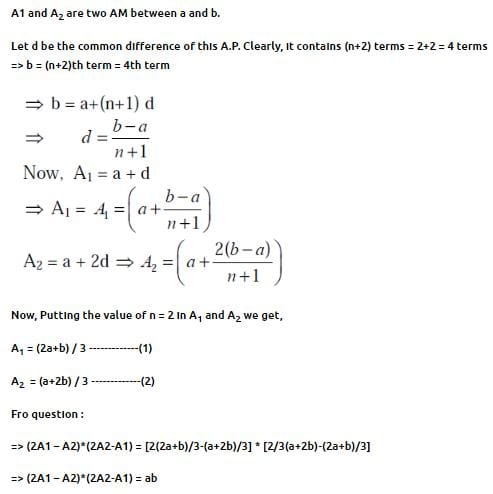Practice Test: Arithmetic Progressions - Question 22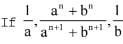are in A.P., then n is equal to

Practice Test: Arithmetic Progressions - Question 23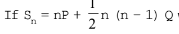where Sn denotes the sum of the first n terms of an A.P., then the common difference of the A.P. is

Practice Test: Arithmetic Progressions - Question 24

If a,b,c are positive reals, then least value of (a + b + c) (1/a+1/b+1/c) is :

Practice Test: Arithmetic Progressions - Question 25

The sum of the first four terms of an A.P. is 56. The sum of the last four terms is 112. If its first term is 11, then find the number of terms.

## Mathematics (Maths) Class 10

53 videos|369 docs|138 tests
 Use Code STAYHOME200 and get INR 200 additional OFF Use Coupon Code
Information about Practice Test: Arithmetic Progressions Page
In this test you can find the Exam questions for Practice Test: Arithmetic Progressions solved & explained in the simplest way possible. Besides giving Questions and answers for Practice Test: Arithmetic Progressions, EduRev gives you an ample number of Online tests for practice

## Mathematics (Maths) Class 10

53 videos|369 docs|138 tests